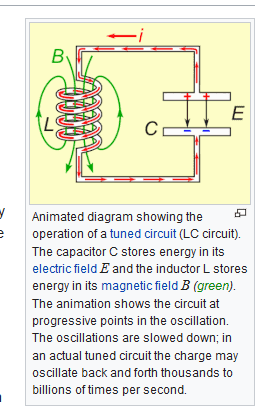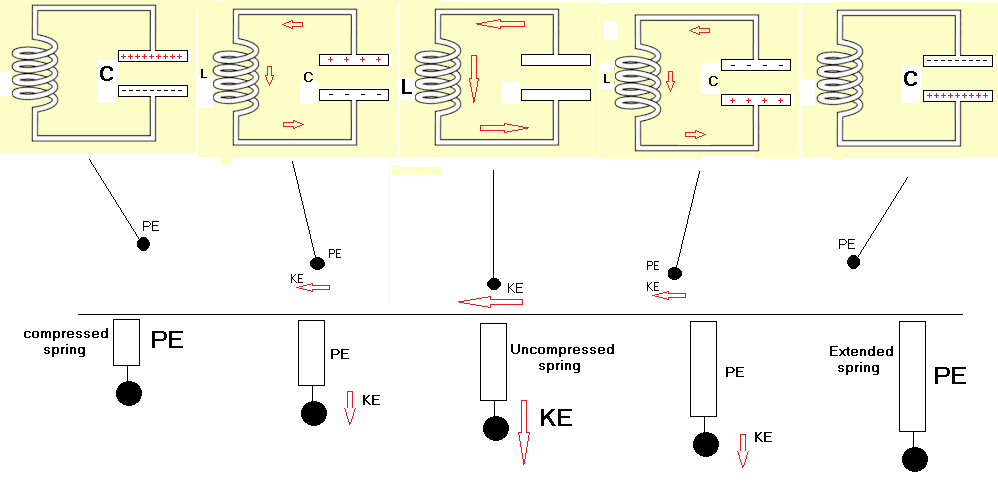# In an LC circuit the current does no drop to 0

Josielle Abdilla
in an LC circuit the current does no drop to 0 but varies sinusoidally. The capacitor is recharged with a different polarization. Why is this so?

Mentor
in an LC circuit the current does no drop to 0 but varies sinusoidally. The capacitor is recharged with a different polarization. Why is this so?
It has to do with the way that the energy stored in the inductor and capacitor are out of phase, so when the capacitor if fully charged one way, it is storing all of the energy in the circuit and the inductor current is zero, and that voltage then causes an increasing current that discharges the capacitor to zero volts, and the inductor stores all of the energy due to the max current that is flowing. That current keeps flowing to charge the capacitor to the other polarity, and the cycle keeps repeating itself.

Have you had basic calculus yet? It's easiest to explain this using the equations for the current and voltage in the capacitor and inductor (and those equations use basic calculus).

https://en.wikipedia.org/wiki/LC_circuit•Josielle Abdilla
Gold Member
I also answered this in your other thread, but I thought I should make a more general comment too.

This circuit is solved with a simple 2nd order differential equation, which I think you haven't studied yet. You'll get to that after a little bit of calculus. That equation appears over and over again in all of the STEM fields. Anytime you hear oscillation, vibration, cyclic, sinusoid, etc, this is likely the root cause of those behaviors.

The solution to this is exactly the same as solving for a mass on a spring (like a car suspension), a pendulum (like how you throw a bowling ball), all musical instruments (like a guitar string, a flute, drums...), a wave in deep water, radio waves, even quantum mechanics and string theory... The list is endless. Physicists call this a harmonic oscillator.

It's a bit hard to explain the LC circuit well without the math; it just isn't very intuitive, like a mass and a spring.

So here's the point: When you get to this subject in your classes, you would do well to recognize that this is a very important problem to learn well; you will see it again and again and again. It is one of those problems that is really complicated at first but as you study more will become very simple as you gain familiarity with it.

•Nugatory, jrmichler, sophiecentaur and 2 others
Homework Helper
Gold Member
Everyone else can explain how you solve 2nd order DE without knowing an answer in advance, but the point made about the commonality of simple harmonic oscillators is useful. Perhaps I can offer a visual?1- Capacitor has electrostatic PE.
- Pendulum has gravitational PE.
2- Voltage starts to drive current through inductor, losing some charge. Current through inductor creates magnetic energy
- Gravity pulls bob down. Bob loses some PE, but gains som KE.
3 - Capacitor has discharged. Current has increased to max. Inductor has max magnetic stored energy.
- Bob at bottom has lost all PE and has max KE.

Does the bob just stop here? Can the current through the inductor just stop here? (Clue: No to both.)
If it causes you any problem, you need to understand more about inductance.

4 - Inductor continues driving current in circuit, losing magnetic energy as it does so. Current starts to charge capacitor.
- Bob keeps moving and rises gaining PE and losing KE.
5 - When all magnetic energy is used, inductor no longer drives current into capacitor. Capacitor has max charge.
- Bob reaches max height and stops. It has max PE and zero KE.

That's half a cycle. The next half cycle is a mirror of this.

If there's no friction of any sort, the pendulum can continue undiminished oscillation. Similarly the LC circuit would continue to oscillate IF there were no losses, but there always are.

The same sort of thing can be done for a Spring - Mass system, where energy exchanges between PE of stretched or compressed spring and KE of mass.

All have a relationship between two stores of energy.

Last edited:
•Lord Crc and zoki85
zoki85
https://www.physicsforums.com/attachments/259381

The same sort of thing can be done for a Spring - Mass system, where energy exchanges between PE of stretched or compressed spring and KE of mass.

All have a relationship between two stores of energy.

In case of L(R)C circuit oscillations, the analogy mechanical system-electrical system works great.
Spring-Mass analogy works even better than pendulum. For the pendulum the undamped oscillation is just aproximate sine (it approaches sine for small angles) while linear spring-mass is pure sine.

•sophiecentaur
Homework Helper
Gold Member
Yes. I first thought of that, but drawing springs is harder than drawing strings!
But you're right, it's a better analogy.

Edit: I've given up tying to draw the springs, just used boxes! So I've updated the diagram.
But it I didn't like the look of it horizontally, so it is vertical. I just hope people won't worry about gravity. It all works out the same, but people might get into a fuss about different sorts of PE.
Any further suggestions welcome.

Last edited:
•sophiecentaur
•